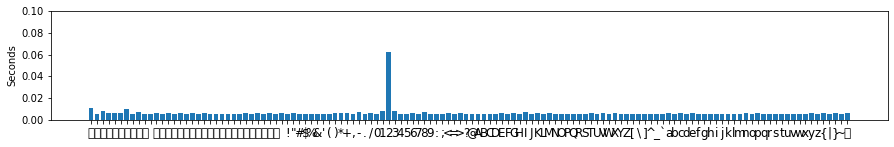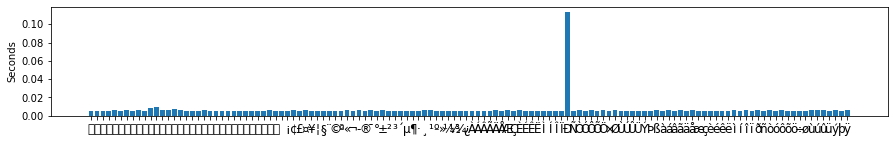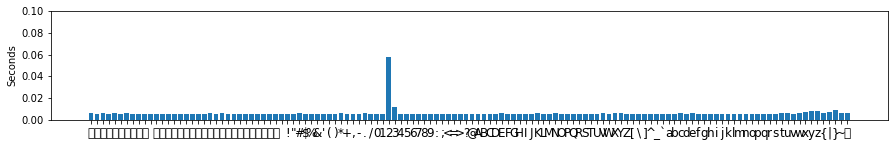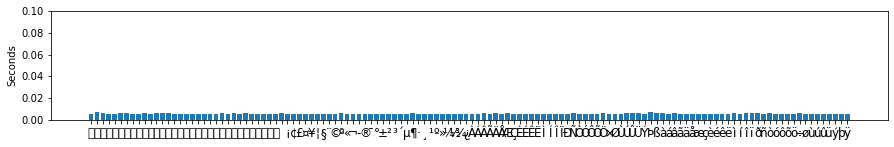# Cryptopals Set 4

### Stream Crypto and Randomness

SPOILER WARNING: Try the cryptopals crypto challenges first!

Set 4 of cryptopals! We are warned that people drop out after this set so let’s make the most of it.

Notes for previous sets:

In :
from binascii import unhexlify, b2a_base64, a2b_base64, hexlify
from base64 import b64decode
from Crypto.Cipher import AES
import random
import string
from itertools import islice
from os.path import commonprefix
import struct
import matplotlib.pyplot as plt
import requests
import numpy as np
from time import time
import warnings
warnings.filterwarnings('ignore')


First, some functions we’ll need from the other sets

In :
def generate_random_bytes(num_bytes: int) -> bytes:
return bytes([random.randint(0, 255) for _ in range(num_bytes)])

def fixed_xor(buffer1: bytes, buffer2: bytes) -> bytes:
return bytes([(b1 ^ b2) for b1, b2 in zip(buffer1, buffer2)])

def aes_in_ecb_mode(byte_string: bytes, key: bytes, encrypt: bool = False) -> bytes:
cipher = AES.new(key, AES.MODE_ECB)
if encrypt:
return cipher.encrypt(byte_string)
else:
return cipher.decrypt(byte_string)

def pkcs7_padding(byte_string: bytes, block_length: int) -> bytes:
num_to_pad = block_length - (len(byte_string) % block_length)
return byte_string + bytes([num_to_pad]) * num_to_pad

def cbc_mode(byte_string: bytes,
key: bytes,
initialization_vector: bytes,
encrypt: bool = True) -> bytes:
if encrypt:
previous_block = initialization_vector
cipher_text = b''
for i in range(0, len(byte_string), len(key)):
plain_text = fixed_xor(pkcs7_padding(byte_string[i: i + len(key)], len(key)),
previous_block)
previous_block = aes_in_ecb_mode(plain_text, key, encrypt=True)
cipher_text += previous_block
return cipher_text
else:
previous_block = initialization_vector
plain_text = b''
for i in range(0, len(byte_string), len(key)):
cipher_text = byte_string[i: i + len(key)]
plain_text += fixed_xor(aes_in_ecb_mode(cipher_text, key, encrypt=False), previous_block)
previous_block = cipher_text
return plain_text

def ctr_keystream(key: bytes, nonce: int):
counter = 0
nonce_bytes = nonce.to_bytes(BLOCK_SIZE // 2, byteorder='little')
while True:
counter_bytes = counter.to_bytes(BLOCK_SIZE // 2, byteorder='little')
yield from aes_in_ecb_mode(nonce_bytes + counter_bytes,
key, encrypt=True)
counter += 1

def ctr_mode(byte_string: bytes, key: bytes, nonce: int) -> bytes:
if len(byte_string) == 0:
return b''
return fixed_xor(byte_string, ctr_keystream(key, nonce))

In :
def bytes_to_str(byte_list: bytes) -> str:
return "".join(filter(lambda x: x in string.printable, "".join(map(chr, byte_list))))

In :
BLOCK_SIZE = 16
GLOBAL_KEY = generate_random_bytes(BLOCK_SIZE)
NONCE = 42


## Challenge 25 - Break “random access read/write” AES CTR¶

Turns out the data for this question is the same ‘YELLOW SUBMARINE’-encoded data from Set 1 Challenge 7.

In :
byte_string = b''.join([a2b_base64(line.strip()) for line in open("data/crypto/25.txt").readlines()])
plaintext = aes_in_ecb_mode(byte_string, b'YELLOW SUBMARINE', encrypt=False)
for line in bytes_to_str(plaintext).split("\n")[:10]:
print(line)

I'm back and I'm ringin' the bell
A rockin' on the mike while the fly girls yell
In ecstasy in the back of me
Well that's my DJ Deshay cuttin' all them Z's
Hittin' hard and the girlies goin' crazy
Vanilla's on the mike, man I'm not lazy.

I'm lettin' my drug kick in
It controls my mouth and I begin
To just let it flow, let my concepts go


Let’s encrypt this song in CTR mode with our random key.

In :
ciphertext = ctr_mode(plaintext, GLOBAL_KEY, nonce=NONCE)


Now, we need to write a function that lets us “seek” into the ciphertext, decrypt, and re-encrypt with different plaintext.

In :
def ctr_edit(ciphertext, offset, newtext):
keystream = islice(ctr_keystream(GLOBAL_KEY, NONCE), offset, offset + len(newtext))
return ciphertext[:offset] + fixed_xor(newtext, keystream) + ciphertext[offset + len(newtext):]

In :
example = ctr_edit(ciphertext, 10, b"look I'm editing this")
print([x for x in example[:20]])
print([x for x in ciphertext[:20]]) # changes from 10 onwards

[150, 92, 217, 148, 156, 223, 19, 246, 8, 108, 240, 109, 173, 101, 120, 238, 45, 116, 235, 110]
[150, 92, 217, 148, 156, 223, 19, 246, 8, 108, 242, 102, 226, 71, 127, 202, 42, 107, 162, 101]


So, as we know from Set 3 Challenge 19 and 20, given ciphertext byte $C_i$ and plaintext byte $P_i$, we can recover keystream byte $K_i$ with: $$C_i \oplus P_i = K_i$$

Since we have control over the plaintext that we input, if we just give it null bytes then we get the keystream right away

In :
real_keystream = bytes(list(islice(ctr_keystream(GLOBAL_KEY, NONCE), 0, len(ciphertext))))

In :
predicted_keystream = ctr_edit(ciphertext, 0, bytes(*len(ciphertext)))

In :
real_keystream == predicted_keystream

Out:
True

We have the ciphertext, we have the keystream, and we have good old invertible XOR. $$C_i \oplus K_i = P_i$$

In :
predicted_plaintext = fixed_xor(ciphertext, predicted_keystream)

In :
for line in bytes_to_str(predicted_plaintext).split("\n")[:10]:
print(line)

I'm back and I'm ringin' the bell
A rockin' on the mike while the fly girls yell
In ecstasy in the back of me
Well that's my DJ Deshay cuttin' all them Z's
Hittin' hard and the girlies goin' crazy
Vanilla's on the mike, man I'm not lazy.

I'm lettin' my drug kick in
It controls my mouth and I begin
To just let it flow, let my concepts go


## Challenge 26 - CTR bitflipping¶

Let’s re-write the CTC bitflipping functions from Set 2 Challenge 16 but with CTR mode.

In :
def ctr_bitflipping(byte_string: bytes, encrypt=True)->bytes:
if encrypt:
prefix = b'comment1=cooking%20MCs;userdata='
suffix = b';comment2=%20like%20a%20pound%20of%20bacon'
input_string = (prefix + byte_string + suffix).replace(b';', b'";"').replace(b'=', b'"="')
return ctr_mode(input_string, GLOBAL_KEY, NONCE)
else:
return ctr_mode(byte_string, GLOBAL_KEY, NONCE)

def ctr_bitflipping_check(byte_string: bytes)->bool:
decrypted_string = ctr_bitflipping(byte_string, encrypt=False)
if b';admin=true;' in decrypted_string:
return True
else:
return False


Our task is to somehow insert the string “;admin=true;” into the byte string, even though the encryptor comments out the ‘;’s and ‘=’s. As before, let’s start with encrypting ‘xadminxtruex’ and then seeing how we can convert the ‘x’s to ‘;’s and ‘=’s.

In :
byte_string = b'xadminxtruex'
ciphertext = ctr_bitflipping(byte_string)


We have $C_i$ and $P_i$ for these specific letters so we can find the respective $K_i$s. The only problem is that we don’t know the $i$s, since we have no idea what the prefixes and suffixes added by the encryptor are. Let’s find out the length of the prefix, by checking how the encryptor behaves on two different inputs (since CTR doesn’t pad, we don’t have to bother with all that block size business):

In :
len_prefix = len(commonprefix([ctr_bitflipping(b''),
ctr_bitflipping(b'A')]))

In :
len('comment1"="cooking%20MCs";"userdata"="') == len_prefix

Out:
True

We need to change our byte_string at indices 0, 6, and 11, so let’s get the keystream values there.

In :
K_0 = ciphertext[len_prefix + 0] ^ ord('x')
K_6 = ciphertext[len_prefix + 6] ^ ord('x')
K_11 = ciphertext[len_prefix + 11] ^ ord('x')


Now we get the corresponding $C_i^*$s, i.e. what we need to change the ciphertext to for the decryptor to return what we want, and put them in where they belong.

In :
C_0 = K_0 ^ ord(';')
C_6 = K_6 ^ ord('=')
C_11 = K_11 ^ ord(';')

ciphertext = bytearray(ciphertext)
ciphertext[len_prefix + 0] = C_0
ciphertext[len_prefix + 6] = C_6
ciphertext[len_prefix + 11] = C_11
ciphertext = bytes(ciphertext)


Did it work?

In :
ctr_bitflipping_check(ciphertext)

Out:
True

## Challenge 27 - Recover the key from CBC with IV=Key¶

This exercise is meant to demonstrate the pitfalls of repurposing the key as the initialization vector in CBC mode. We’ll rewrite our Set 2 Challenge 16 function to do that.

In :
def cbc_url_encryptor(byte_string: bytes, encrypt=True)->bytes:
if encrypt:
prefix = b'comment1=cooking%20MCs;userdata='
suffix = b';comment2=%20like%20a%20pound%20of%20bacon'
input_string = (prefix + byte_string + suffix).replace(b';', b'";"').replace(b'=', b'"="')
return cbc_mode(pkcs7_padding(input_string, BLOCK_SIZE), GLOBAL_KEY, GLOBAL_KEY, encrypt=True)
else:
return cbc_mode(byte_string, GLOBAL_KEY, GLOBAL_KEY, encrypt=False)


Writing the shady ASCII compliance function:

In :
def is_ascii_compliant(text):
return all(x < 128 for x in text)

def cbc_url_encryptor_check(byte_string:bytes)->bool:
decrypted_string = cbc_url_encryptor(byte_string, encrypt=False)
if not is_ascii_compliant(decrypted_string):
raise ValueError(f"Not ASCII compliant", decrypted_string)

In :
byte_string = b"high ASCII here?"
ciphertext = cbc_url_encryptor(byte_string)
try:
cbc_url_encryptor_check(ciphertext)
print("No high ASCII")
except ValueError as e:
print(e)

No high ASCII

In :
byte_string += bytes()
ciphertext = cbc_url_encryptor(byte_string)
try:
cbc_url_encryptor_check(ciphertext)
print("No high ASCII")
except ValueError as e:
print(e)

('Not ASCII compliant', b'comment1"="cooking%20MCs";"userdata"="high ASCII here?\x81";"comment2"="%20like%20a%20pound%20of%20bacon\x0b\x0b\x0b\x0b\x0b\x0b\x0b\x0b\x0b\x0b\x0b')


Okay, time to find the key.

We’ve been using a block size of 16 everywhere, but let’s assume we don’t know the block size and find it again, by adding one character at a time till the output length changes (since CBC pads, it jumps all at once to the next block size):

In :
MAX_SIZE = 64
def find_block_size(encryptor):
length_output = len(encryptor(b'A'*0))
for i in range(1, MAX_SIZE):
new_length_output = len(encryptor(b'A'*i))
block_size = new_length_output - length_output
if block_size != 0:
break
length_output = new_length_output
return block_size

cbc_url_block_size = find_block_size(cbc_url_encryptor)

In :
cbc_url_block_size

Out:
16
In :
plaintext = generate_random_bytes(cbc_url_block_size * 3)
ciphertext = cbc_url_encryptor(plaintext)

In :
# Number of blocks taken up by the prefix:
num_prefix_blocks = len(commonprefix([cbc_url_encryptor(b''),
cbc_url_encryptor(b'A')])) // cbc_url_block_size + 1

In :
# Number of extra letters to add such that
# the prefix + extra takes up a whole number of blocks
encrypted_strings = [cbc_url_encryptor(b'A'*0)]
min_addition = None
for i in range(1, cbc_url_block_size):
encrypted_strings.append(cbc_url_encryptor(b'A'*i))
length_common_prefix = len(commonprefix(encrypted_strings))
if length_common_prefix == num_prefix_blocks * cbc_url_block_size:
min_addition = i-1
break
encrypted_strings = [encrypted_strings[-1]]
assert min_addition is not None

In :
len_prefix = num_prefix_blocks * cbc_url_block_size - min_addition
len('comment1"="cooking%20MCs";"userdata"="') == len_prefix

Out:
True
In :
ciphertext = ciphertext[len_prefix: cbc_url_block_size + len_prefix] + \
bytes( * cbc_url_block_size) + \
ciphertext[len_prefix: cbc_url_block_size + len_prefix]

In :
try:
cbc_url_encryptor_check(ciphertext)
except ValueError as e:
modified_plaintext = e.args


Remembering CBC mode:

$$P_1 = decrypt(C_1) \oplus iv$$$$P_3 = decrypt(C_1) \oplus C_2 = decrypt(C_1)$$

since $C_2 = 0$ after we messed with it.

$$P_1 = P_3 \oplus iv$$$$=> iv = P_1 \oplus P_3$$
In :
IV = fixed_xor(modified_plaintext[:cbc_url_block_size],
modified_plaintext[2*cbc_url_block_size:])


Cracking the IV wouldn’t normally be the end of the world, but this time we were lax and used the IV as the key. Shame on us.

In :
IV == GLOBAL_KEY

Out:
True

## Challenge 28- Implement a SHA-1 keyed MAC¶

Wikipedia again with its beautiful pseudocode.

In :
MASK = 0xffffffff

def sha1(message: bytes, h0=0x67452301, h1=0xEFCDAB89, h2=0x98BADCFE, h3=0x10325476, h4=0xC3D2E1F0):
message += get_sha1_padding(len(message))
return sha1_no_padding(message, h0, h1, h2, h3, h4)

def get_sha1_padding(m1: int) -> bytes:
# append the bit '1' to the message
padding = b'\x80'
# append 0 <= k < 512 bits '0', such that the resulting message length in bits
# is congruent to 448 (mod 512). In bytes this is 56 (mod 64)
padding += b'\x00' * ((56 - (m1 + 1) % 64) % 64)
# append ml, the original message length, as a 64-bit big-endian integer.
# m1 * 8 = length in bits
padding += struct.pack(b'>Q', m1 * 8)
return padding

def left_rotate(x, y):
return ((x << y) | (x >> (32 - y))) & MASK

def chunks(message, chunk_size=64):
for i in range(0, len(message), chunk_size):
yield message[i: i+chunk_size]

def sha1_no_padding(message: bytes, h0=0x67452301, h1=0xEFCDAB89, h2=0x98BADCFE, h3=0x10325476, h4=0xC3D2E1F0):
for chunk in chunks(message):
w =  * 80
# break chunk into sixteen 32-bit big-endian words w[i], 0 ≤ i ≤ 15
for i in range(16):
w[i] = struct.unpack(b'>I', chunk[i * 4:i * 4 + 4])
# Message schedule: extend the sixteen 32-bit words into eighty 32-bit words:
for i in range(16, 80):
w[i] = (left_rotate(w[i - 3] ^ w[i - 8] ^ w[i - 14] ^ w[i - 16], 1)) & MASK
a, b, c, d, e = h0, h1, h2, h3, h4
for i in range(80):
if 0 <= i <= 19:
f = d ^ (b & (c ^ d)) # alternative 1
k = 0x5A827999
elif 20 <= i <= 39:
f = b ^ c ^ d
k = 0x6ED9EBA1
elif 40 <= i <= 59:
f = (b & c) | (b & d) | (c & d)
k = 0x8F1BBCDC
elif 60 <= i <= 79:
f = b ^ c ^ d
k = 0xCA62C1D6
a, b, c, d, e = ((left_rotate(a, 5) + f + e + k + w[i]) & MASK,
a, left_rotate(b, 30), c, d)
h0 = (h0 + a) & MASK
h1 = (h1 + b) & MASK
h2 = (h2 + c) & MASK
h3 = (h3 + d) & MASK
h4 = (h4 + e) & MASK
return b''.join(h.to_bytes(4, byteorder='big') for h in [h0, h1, h2, h3, h4])

In :
hexlify(sha1(b"The quick brown fox jumps over the lazy dog")) == b"2fd4e1c67a2d28fced849ee1bb76e7391b93eb12"

Out:
True
In :
hexlify(sha1(b"The quick brown fox jumps over the lazy cog")) == b"de9f2c7fd25e1b3afad3e85a0bd17d9b100db4b3"

Out:
True
In :
def MAC(message: bytes, key=GLOBAL_KEY,
h0=0x67452301, h1=0xEFCDAB89,
h2=0x98BADCFE, h3=0x10325476,
h4=0xC3D2E1F0) -> bytes:
return sha1(key + message, h0, h1, h2, h3, h4)

In :
mac = MAC(b"The quick brown fox jumps over the lazy dog")


Changing the message changes the MAC, even if you just change one letter

In :
MAC(b"The quick brown fox jumps over the lazy cog") == mac

Out:
False

Without the key you can’t get back the MAC even with the same message

In :
MAC(b"The quick brown fox jumps over the lazy cog", key=b"") == mac

Out:
False

## Challenge 29 - Break a SHA-1 keyed MAC using length extension¶

We’re back to our wily admin tricks.

I went back and changed the SHA-1 implementation above to have the padding as a separate function and to give h0-h4 as parameters (I know you can’t see me do this, I’m just rambling out loud).

In :
original_message = b"comment1=cooking%20MCs;userdata=foo;comment2=%20like%20a%20pound%20of%20bacon"
mac = MAC(original_message)


We’d like to append a new message to the original one and get a valid MAC for this (original + new) message without going through the trouble of stealing someone’s key.

In :
new_message = b";admin=true;"


The trick here is that SHA-1 processes messages 512 bits (64 bytes) at a time, with each chunk updating the state of h0-h4. These are then concatenated and returned as the hash. So after MAC-ing the original_message, we can break up the returned hash into h0-h4, start off a new SHA-1 instance initialized with these values, and feed it in extra stuff. This extra stuff would then be hashed as if it was a part of the original message all along.

In :
h0 = int.from_bytes(mac[0:4], byteorder='big')
h1 = int.from_bytes(mac[4:8], byteorder='big')
h2 = int.from_bytes(mac[8:12], byteorder='big')
h3 = int.from_bytes(mac[12:16], byteorder='big')
h4 = int.from_bytes(mac[16:20], byteorder='big')


Of course, the MAC hashes the key + original_message, and the hash itself is done on the padded version of this (the so-called glue padding in the question). We don’t know the key though so we’ll have to guess at its length until we crack it.

And, though we’re only forging the hash function for a small new_message, we want it to behave as if it’s acting on the whole (original + new) message so we have to pad it as if it has the whole thing.

In :
len_message = len(original_message) + len(new_message)
for key_length in range(64):
glue_padding = get_sha1_padding(key_length + len(original_message))
new_padding = get_sha1_padding(key_length + len_message + len(glue_padding))
new_mac = sha1_no_padding(new_message + new_padding, h0, h1, h2, h3, h4)
if new_mac == MAC(original_message + glue_padding + new_message):
print(f"Success! Key length {key_length}")

Success! Key length 16


## Challenge 30 - Break an MD4 keyed MAC using length extension¶

The wiki page doesn’t have pseudocode this time :( But I found some on this practical cryptography website. Hmm, it seems to use exactly the same padding very similar but not exactly the same padding as SHA-1 (you append the little-endian version of the bit length instead of big-endian).

In :
def md4(message: bytes, h0=0x67452301, h1=0xefcdab89, h2=0x98badcfe, h3=0x10325476):
message = message + get_md4_padding(len(message))
return md4_no_padding(message, h0, h1, h2, h3)

def get_md4_padding(m1: int) -> bytes:
padding = b'\x80'
padding += b'\x00' * ((56 - (m1 + 1) % 64) % 64)
padding += struct.pack(b'<Q', m1 * 8) # THIS LINE IS DIFFERENT FROM SHA-1
return padding

def md4_no_padding(message: bytes, h0=0x67452301, h1=0xefcdab89, h2=0x98badcfe, h3=0x10325476):
_F = lambda x, y, z: ((x & y) | (~x & z))
_G = lambda x, y, z: ((x & y) | (x & z) | (y & z))
_H = lambda x, y, z: (x ^ y ^ z)
round_1 = lambda w, x, y, z, k, s: left_rotate((w + _F(x, y, z) + X[k]) & MASK, s)
round_2 = lambda w, x, y, z, k, s: left_rotate((w + _G(x, y, z) + X[k] + 0x5a827999) & MASK, s)
round_3 = lambda w, x, y, z, k, s: left_rotate((w + _H(x, y, z) + X[k] + 0x6ed9eba1) & MASK, s)

for chunk in chunks(message):
X = list(struct.unpack('<' + 'I' * 16, chunk))
a, b, c, d = h0, h1, h2, h3

# Round 1
a = round_1(a, b, c, d, 0, 3)
d = round_1(d, a, b, c, 1, 7)
c = round_1(c, d, a, b, 2, 11)
b = round_1(b, c, d, a, 3, 19)

a = round_1(a, b, c, d, 4, 3)
d = round_1(d, a, b, c, 5, 7)
c = round_1(c, d, a, b, 6, 11)
b = round_1(b, c, d, a, 7, 19)

a = round_1(a, b, c, d, 8, 3)
d = round_1(d, a, b, c, 9, 7)
c = round_1(c, d, a, b, 10, 11)
b = round_1(b, c, d, a, 11, 19)

a = round_1(a, b, c, d, 12, 3)
d = round_1(d, a, b, c, 13, 7)
c = round_1(c, d, a, b, 14, 11)
b = round_1(b, c, d, a, 15, 19)

# Round 2
a = round_2(a, b, c, d, 0, 3)
d = round_2(d, a, b, c, 4, 5)
c = round_2(c, d, a, b, 8, 9)
b = round_2(b, c, d, a, 12, 13)

a = round_2(a, b, c, d, 1, 3)
d = round_2(d, a, b, c, 5, 5)
c = round_2(c, d, a, b, 9, 9)
b = round_2(b, c, d, a, 13, 13)

a = round_2(a, b, c, d, 2, 3)
d = round_2(d, a, b, c, 6, 5)
c = round_2(c, d, a, b, 10, 9)
b = round_2(b, c, d, a, 14, 13)

a = round_2(a, b, c, d, 3, 3)
d = round_2(d, a, b, c, 7, 5)
c = round_2(c, d, a, b, 11, 9)
b = round_2(b, c, d, a, 15, 13)

# Round 3
a = round_3(a, b, c, d, 0, 3)
d = round_3(d, a, b, c, 8, 9)
c = round_3(c, d, a, b, 4, 11)
b = round_3(b, c, d, a, 12, 15)

a = round_3(a, b, c, d, 2, 3)
d = round_3(d, a, b, c, 10, 9)
c = round_3(c, d, a, b, 6, 11)
b = round_3(b, c, d, a, 14, 15)

a = round_3(a, b, c, d, 1, 3)
d = round_3(d, a, b, c, 9, 9)
c = round_3(c, d, a, b, 5, 11)
b = round_3(b, c, d, a, 13, 15)

a = round_3(a, b, c, d, 3, 3)
d = round_3(d, a, b, c, 11, 9)
c = round_3(c, d, a, b, 7, 11)
b = round_3(b, c, d, a, 15, 15)

h0 = (h0 + a) & MASK
h1 = (h1 + b) & MASK
h2 = (h2 + c) & MASK
h3 = (h3 + d) & MASK

return struct.pack('<IIII', h0, h1, h2, h3)

In :
hexlify(md4(b"The quick brown fox jumps over the lazy dog")) == b"1bee69a46ba811185c194762abaeae90"

Out:
True
In :
hexlify(md4(b"The quick brown fox jumps over the lazy cog")) == b"b86e130ce7028da59e672d56ad0113df"

Out:
True

Rinse and repeat. Everything’s the same as in the previous challenge except that the byteorder becomes ‘little’

In :
def MAC_md4(message: bytes, key=GLOBAL_KEY, h0=0x67452301, h1=0xefcdab89, h2=0x98badcfe, h3=0x10325476) -> bytes:
return md4(key + message, h0, h1, h2, h3)

original_message = b"comment1=cooking%20MCs;userdata=foo;comment2=%20like%20a%20pound%20of%20bacon"
mac = MAC_md4(original_message)

new_message = b";admin=true;"

h0 = int.from_bytes(mac[0:4], byteorder='little')
h1 = int.from_bytes(mac[4:8], byteorder='little')
h2 = int.from_bytes(mac[8:12], byteorder='little')
h3 = int.from_bytes(mac[12:16], byteorder='little')

for key_length in range(64):
glue_padding = get_md4_padding(key_length + len(original_message))
new_padding = get_md4_padding(len(new_message) + \
key_length +  len(original_message) + len(glue_padding))
new_mac = md4_no_padding(new_message + new_padding, h0, h1, h2, h3)
if new_mac == MAC_md4(original_message + glue_padding + new_message):
print(f"Success! Key length {key_length}")

Success! Key length 16


## Challenge 31 - Implement and break HMAC-SHA1 with an artificial timing leak¶

You know the drill: to Wikipedia!

In :
def hmac(message: bytes, key: bytes = GLOBAL_KEY,
hash_fn=sha1,
block_size=64, output_size=20):
if len(key) > block_size:
key = hash_fn(key)
if len(key) < block_size:
key = key + b'\x00' * (block_size - len(key))
o_key_pad = fixed_xor(key, bytes.fromhex("5c") * block_size)
i_key_pad = fixed_xor(key, bytes.fromhex("36") * block_size)
return hash_fn(o_key_pad + hash_fn(i_key_pad + message))

In :
hexlify(hmac(b"The quick brown fox jumps over the lazy dog", key=b"key")) == b"de7c9b85b8b78aa6bc8a7a36f70a90701c9db4d9"

Out:
True

I’m going to use the recommended web.py to build the framework. I’ve put the below in a file called app.py along with the hmac and sha1 functions and the GLOBAL_KEY from above.

In [ ]:
import web
from time import sleep
from binascii import hexlify

class test:
def GET(self):
user_data = web.input(file=None, signature=None)
assert user_data.file is not None and user_data.signature is not None
if insecure_compare(file, signature):
return web.OK()
else:
return web.InternalError("Invalid MAC")

def insecure_compare(file, signature):
file_mac = hexlify(hmac(file.encode("utf-8"), key=GLOBAL_KEY))
signature = signature.encode("utf-8")
for i in range(len(file_mac)):
if i >= len(signature) or signature[i] != file_mac[i]:
return False
sleep(0.05)
return True

if __name__ == "__main__":
urls = ('/test', test_page)
app = web.application(urls, globals())
app.run()


For a filename of “foo” the server is expecting the signature:

In :
hexlify(hmac(b"foo"))

Out:
b'209fca41418ae2b699a67882ac549ce19c6cc5ec'
In :
payload = {'file': 'foo', 'signature': '209fca41418ae2b699a67882ac549ce19c6cc5ec'}
response = requests.get('http://localhost:8080/test', params=payload)
response

Out:
Response 

So the trick here is to time the response for different inputs and discover each correct byte one at a time. Let’s see how the distribution of time is for different variations of the first byte of the signature:

In :
times = np.zeros(256)
for b in range(256):
payload = {'file': 'foo', 'signature': chr(b)}
response = requests.get('http://localhost:8080/test', params=payload)
times[b] = response.elapsed.total_seconds()

In :
def plot_times(times):
plt.figure(figsize=(15,2))
plt.bar(range(128), times[:128])
plt.xticks(range(128), [chr(b) for b in range(128)], fontsize="large")
plt.yticks(np.arange(0, 0.12, 0.02))
plt.ylabel("Seconds")
plt.show()
plt.figure(figsize=(15,2))
plt.bar(range(128), times[128:])
plt.xticks(range(128), [chr(b) for b in range(128, 256)], fontsize="large")
plt.yticks(np.arange(0, 0.12, 0.02))
plt.ylabel("Seconds")
plt.show()

plot_times(times)There’s definitely a peak at 2 but response times can be a bit noisy so there’s also another peak (and since we don’t actually know that the sleep time is 0.05 seconds, it’s hard to say which is the right peak). So we’ll repeat the process a bunch of times and take the median to smoothen out the noise.

In :
times = np.zeros((4, 256))
for r in range(4):
for b in range(256):
payload = {'file': 'foo', 'signature': chr(b)}
response = requests.get('http://localhost:8080/test', params=payload)
times[r, b] = response.elapsed.total_seconds()
times = np.median(times, axis=0)
plot_times(times)Okay, time to encapsulate this into a function.

In :
def timing_attack(file, num_rounds=3):
start_time = time()

signature = ''
payload = {'file': file}
current_times = np.zeros((num_rounds, 256))

while True:
for r in range(num_rounds):
for candidate in range(256):
payload['signature'] = f"{signature}{chr(candidate)}"
response = requests.get('http://localhost:8080/test', params=payload)
if response.ok:
return payload['signature']
current_times[r, candidate] = response.elapsed.total_seconds()
correct_candidate = np.argmax(np.median(current_times, axis=0))
signature = f"{signature}{chr(correct_candidate)}"
print(f"Time elapsed: {time() - start_time:.2f} s; Current signature: {signature}")

In :
timing_attack("foo")

Time elapsed: 6.85 s; Current signature: 2
Time elapsed: 58.31 s; Current signature: 20
Time elapsed: 150.67 s; Current signature: 209
Time elapsed: 283.72 s; Current signature: 209f
Time elapsed: 457.67 s; Current signature: 209fc
Time elapsed: 671.51 s; Current signature: 209fca
Time elapsed: 925.86 s; Current signature: 209fca4
Time elapsed: 1221.55 s; Current signature: 209fca41
Time elapsed: 1557.02 s; Current signature: 209fca414
Time elapsed: 1961.81 s; Current signature: 209fca4141
Time elapsed: 2408.98 s; Current signature: 209fca41418
Time elapsed: 3977.50 s; Current signature: 209fca41418a
Time elapsed: 4471.69 s; Current signature: 209fca41418ae
Time elapsed: 5011.57 s; Current signature: 209fca41418ae2
Time elapsed: 5595.84 s; Current signature: 209fca41418ae2b
Time elapsed: 6218.86 s; Current signature: 209fca41418ae2b6
Time elapsed: 6881.63 s; Current signature: 209fca41418ae2b69
Time elapsed: 7584.56 s; Current signature: 209fca41418ae2b699
Time elapsed: 8327.81 s; Current signature: 209fca41418ae2b699a
Time elapsed: 9112.03 s; Current signature: 209fca41418ae2b699a6
Time elapsed: 9942.72 s; Current signature: 209fca41418ae2b699a67
Time elapsed: 10852.54 s; Current signature: 209fca41418ae2b699a678
Time elapsed: 11804.74 s; Current signature: 209fca41418ae2b699a6788
Time elapsed: 12800.71 s; Current signature: 209fca41418ae2b699a67882
Time elapsed: 13844.81 s; Current signature: 209fca41418ae2b699a67882a
Time elapsed: 14926.29 s; Current signature: 209fca41418ae2b699a67882ac
Time elapsed: 16051.66 s; Current signature: 209fca41418ae2b699a67882ac5
Time elapsed: 17219.45 s; Current signature: 209fca41418ae2b699a67882ac54
Time elapsed: 18429.87 s; Current signature: 209fca41418ae2b699a67882ac549
Time elapsed: 19683.09 s; Current signature: 209fca41418ae2b699a67882ac549c
Time elapsed: 20979.95 s; Current signature: 209fca41418ae2b699a67882ac549ce
Time elapsed: 22319.65 s; Current signature: 209fca41418ae2b699a67882ac549ce1
Time elapsed: 23702.37 s; Current signature: 209fca41418ae2b699a67882ac549ce19
Time elapsed: 25127.76 s; Current signature: 209fca41418ae2b699a67882ac549ce19c
Time elapsed: 26595.78 s; Current signature: 209fca41418ae2b699a67882ac549ce19c6
Time elapsed: 28106.77 s; Current signature: 209fca41418ae2b699a67882ac549ce19c6c
Time elapsed: 29660.73 s; Current signature: 209fca41418ae2b699a67882ac549ce19c6cc
Time elapsed: 31257.14 s; Current signature: 209fca41418ae2b699a67882ac549ce19c6cc5
Time elapsed: 32898.04 s; Current signature: 209fca41418ae2b699a67882ac549ce19c6cc5e

Out:
'209fca41418ae2b699a67882ac549ce19c6cc5ec'

Takes a while but hackers have nothing but time I suppose.

## Challenge 32 - Break HMAC-SHA1 with a slightly less artificial timing leak¶

Hmm, I feel like this one just needs to increase the number of rounds. Another idea is to keep track of multiple possible signatures at once until each one has enough characters for the stacked time to be accurately measurable.

So that’s Set 4.

For comments, click the arrow at the top right corner.